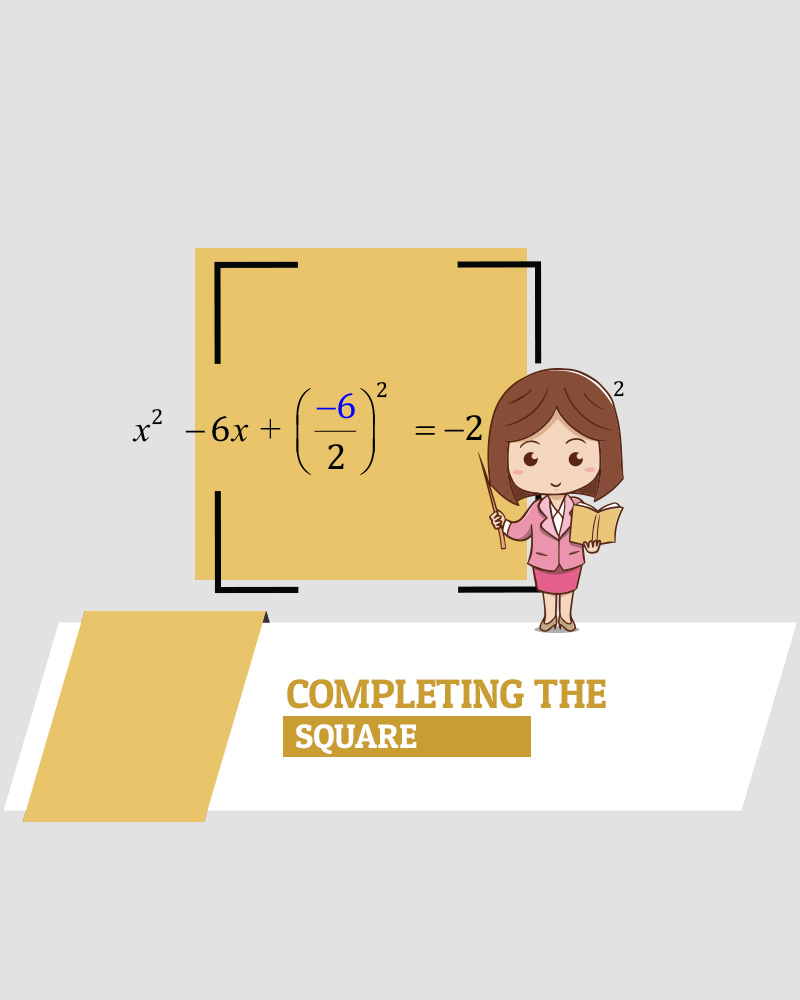# Completing the Square

Quadratic functions have the standard form of ax^2 +bx +c  where a, b, and c are known values.

Changing the form to a(x+h)^2 +k is called the vertex form by completing the square.  There are three steps.

• Step 1: Isolate the constant, c
• Step 2: Make the leading coefficient a =1 by dividing the first two terms by a.
• Step 3: Add and subtract half the coefficient of the middle term squared